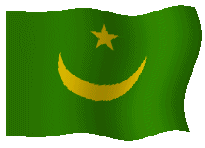# Waypoints### Waypoints Mauritanië

Nouâdihibou

 Lat: 20.900 Long: 17.067 (represented in decimal degrees and fractions of degrees) Lat: 20 54 N Long: 017 04 W (represented in degrees minutes direction)

Nouâdhibou, Râs (Cap Blanc)

 Lat: 20 46 N Long: 017 03 W (represented in degrees minutes direction) Lat: 20.767 Long: 17.050 (represented in decimal degrees and fractions of degrees)

Rosso Senegal river)

 Lat: 16 29 N Long: 015 53 W (represented in degrees minutes direction) Lat: 16.483 Long: 15.883 (represented in decimal degrees and fractions of degrees)

Atar

 Lat: 20 32 N Long: 013 08 W (represented in degrees minutes direction) Lat: 20.533 Long: 13.133 (represented in decimal degrees and fractions of degrees)

Choum

 Lat: 21 18 N Long: 012 58 W (represented in degrees minutes direction) Lat: 21.300 Long: 12.967 (represented in decimal degrees and fractions of degrees)

Chinguetti

 Lat: 20 25 N Long: 012 24 W (represented in degrees minutes direction) Lat: 20.417 Long: 12.400 (represented in decimal degrees and fractions of degrees)

 Lat: 20 58 N Long: 011 30 E (represented in degrees minutes direction) Lat: 20.967 Long: 11.500 (represented in decimal degrees and fractions of degrees)

Akjoujt

 Lat: 19 44 N Long: 014 20 W (represented in degrees minutes direction) Lat: 19.733 Long: 14.333 (represented in decimal degrees and fractions of degrees)

Tidjikja

 Lat: 18 29 N Long: 011 31 W (represented in degrees minutes direction) Lat: 18.483 Long: 11.517 (represented in decimal degrees and fractions of degrees)

Tichitt

 Lat: 18 21 N Long: 009 20 W (represented in degrees minutes direction) Lat: 18.350 Long: 09.333 (represented in decimal degrees and fractions of degrees)

Oualata

 Lat: 17 15 N Long: 006 55 W (represented in degrees minutes direction) Lat: 17.250 Long: 06.917 (represented in decimal degrees and fractions of degrees)

Néma

 Lat: 16 32 N Long: 007 12 W (represented in degrees minutes direction) Lat: 16.533 Long: 07.200 (represented in decimal degrees and fractions of degrees)

Timbédra

 Lat: 16 17 N Long: 008 16 W (represented in degrees minutes direction) Lat: 16.283 Long: 08.267 (represented in decimal degrees and fractions of degrees)

‘Ayoun el ‘Atrous

 Lat: 16 40 N Long: 009 37 W (represented in degrees minutes direction) Lat: 16.667 Long: 09.617 (represented in decimal degrees and fractions of degrees)

Kiffa

 Lat: 16 38 N Long: 011 28 W (represented in degrees minutes direction) Lat: 16.633 Long: 11.467 (represented in decimal degrees and fractions of degrees)

Aleg

 Lat: 17 02 N Long: 013 55 W (represented in degrees minutes direction) Lat: 17.033 Long: 13.917 (represented in decimal degrees and fractions of degrees)

Boutilimit

 Lat: 17 40 N Long: 014 46 W (represented in degrees minutes direction) Lat: 17.667 Long: 14.767 (represented in decimal degrees and fractions of degrees)

Nouakchott

 Lat: 18 09 N Long: 015 58 W (represented in degrees minutes direction) Lat: 18.150 Long: 15.967 (represented in decimal degrees and fractions of degrees)

Kaédi  (Senegal river)

 Lat: 16 12 N Long: 013 32 W (represented in degrees minutes direction) Lat: 16.200 Long: 13.533 (represented in decimal degrees and fractions of degrees)